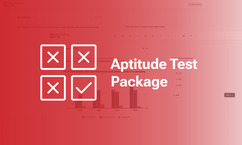Table of content

SHL numerical reasoning tests

# How to Pass SHL Numerical Reasoning Tests? (Free Practice Tests & Guidelines)

Being one of the best-known test publishers, SHL provides evaluation tools for various industries worldwide, including reputed companies like Amazon and Microsoft. Among SHL aptitude assessments, SHL Numerical Reasoning Tests are the most used to filter out candidates in the hiring process.

What’s inside one of the most challenging assessments of SHL? Why is it trusted by thousands of companies in their screening stage? How to nail SHL Numerical Reasoning Tests? This article will give you the answer to all these questions.

## What are SHL numerical reasoning tests?

SHL Numerical Reasoning Tests are among the most common aptitude tests around the world, which evaluate a candidate’s mathematical ability. In other words, you have to analyze, interpret numerical data, and perform quick and accurate calculations throughout the tests.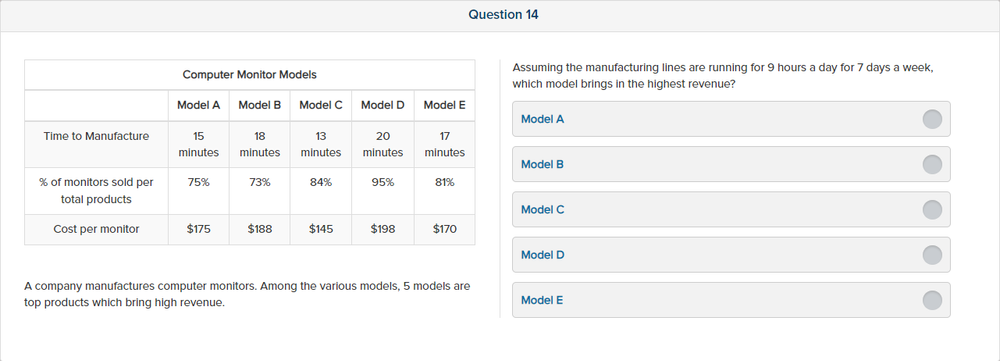Source: SHL

#### Which companies use SHL Numerical Reasoning Tests?

SHL Aptitude Tests, including SHL Numerical Reasoning, are trusted by over 10,000 companies from various industries around the world. Some reputed brand names that use SHL Numerical Reasoning Test come from the top 50% of the Fortune Global 500 and the top 80% of the FTSE 100, such as Amazon, Microsoft, Heineken, or HDFC Bank.

#### What is a good score on SHL Numerical Reasoning Tests?

There is no fixed benchmark for passing SHL Numerical Reasoning Tests. However, there is a “safe” percentile rank for you to pass, typically top 20% of test takers. The pass mark for each recruitment is often determined by the norm group and the number of job vacancies.

#### Can you use a calculator in SHL Numerical Reasoning Tests?

Candidates are permitted to use a calculator in SHL Numerical Reasoning Tests. The only test type that doesn’t allow a calculator is SHL Calculation Test, which is a separate examination and, fortunately, not included in SHL Numerical Reasoning Tests.

#### What is the format of SHL Numerical Reasoning Tests?

There are two versions of SHL Numerical Reasoning Tests, namely:

SHL Verify Numerical Reasoning Test: Use classic multiple-choice questions.
SHL Verify Interactive Numerical Reasoning Test: Use drag/drop format rather than standard multiple-choice inputs.

However, the level of difficulty of the two formats is the same. You need to read the instructions at the beginning of the test to know which one you’ll take.

## SHL Verify vs. SHL Verify Interactive

As mentioned above, when taking the SHL Numerical Reasoning Test, you will be given 1 of 2 versions: Verify and Verify Interactive. So what is the difference between them? Let’s find out through the comparison table below: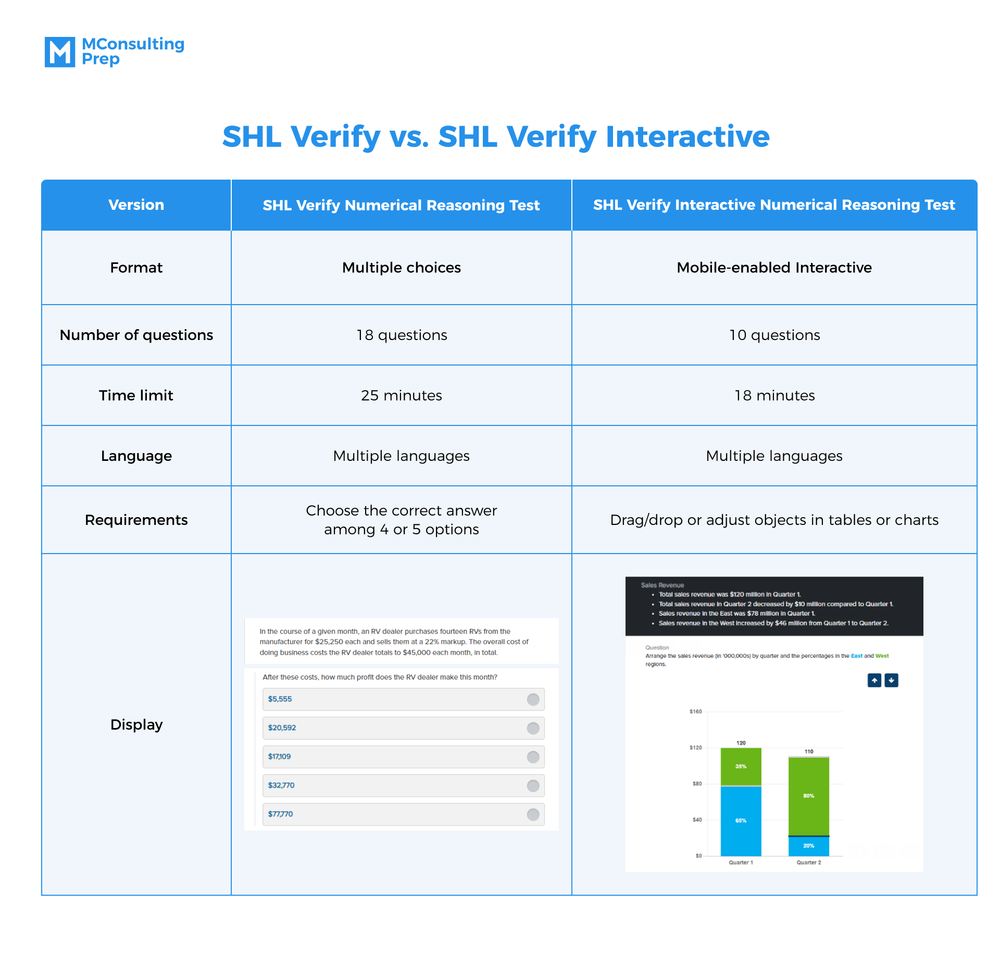## What makes SHL numerical reasoning test so challenging?

SHL Numerical Reasoning Test is considered one of the most taxing SHL aptitude tests due to 3 reasons:

Strict time limits

In the SHL Verify Numerical Reasoning Test, you have to answer 18 questions in 25 minutes, while the interactive test version includes 10 questions within 18 minutes. Therefore, you only have slightly over 1 minute for each question to understand the problem and calculate the correct answer. So besides quick calculation, it is important to have excellent time management skills to solve all the questions on time.

Data complexity

An SHL Numerical Reasoning question can be complicated since there will be various kinds of data you may not know which one to use and how to use it. Moreover, there are many distractions in the question, which aim to test the candidate’s ability to select and process information. Though requiring only basic math operations, the Numerical Reasoning Test involves multiple calculation steps to get the correct answer.

Various skills required

In this assessment, it is crucial to have excellent numeracy ability as a matter of course. However, the SHL Numerical Reasoning Test also requires your reading comprehension skills as the instruction and math problems are often in written format. Moreover, in the Data Interpretation questions, you also need visual reasoning aptitudes to analyze and manipulate graphical and tabular information.

## Ace common question types in SHL numerical reasoning tests

In SHL Numerical Reasoning Tests, you often face 2 common types of questions: Word Problem and Data Interpretation. The next part of this article will show you what to expect and how to master each of these types.

### Word problem

In this question type, you will encounter mathematical questions in written format. Your job is to extract the information from the text and perform arithmetic calculations such as addition, subtraction, multiplication, or division. This aims to measure your numerical reasoning skills and reading comprehension as all the questions are prose.

Question 1: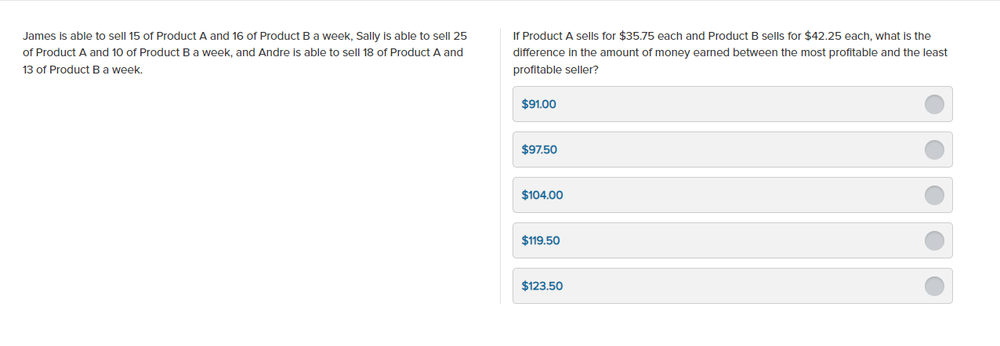Source: SHL

Explanation:
The weekly revenue of James: 15x \$35.75 + 16x \$42.25= \$1212.25
The weekly revenue of Sally: 25x \$35.75 + 10x \$42.25 =\$1316.25
The weekly revenue of Andre: 18x \$35.75 + 13x \$42.25 = \$1192.75
So, the most profitable seller is Sally and the least is Andre.
The difference in the amount of money earned between Sally and Andre is: \$1316.25 – \$1192.75 = \$123.50

Therefore, the correct answer is E.

Word problems can be complicated as they involve reading, which requires you to bridge linguistic information and mathematical expressions. However, we will provide you with some helpful guidelines to ace this challenging question type:

Memorize basic calculations: Word problems can take a lot of time to process written information and convert it into math operations. Therefore, it is crucial to bear in mind basic arithmetic formulas to solve lengthy questions within the time limit.
Translate the problem into mathematical notation: Since reading makes it difficult to understand and follow word problems, you can simplify them by making arithmetic expressions out of the text. In addition, drawing tables and graphs can also be helpful.
Practice common test types: There are several numerical word problems commonly used: time & work, percentages, ratios & proportion, or profit & loss. Therefore, practicing as many test types as possible beforehand to determine specific formulas and techniques for each can help you immensely.

Let’s practice with another word problem from SHL!

Question 2: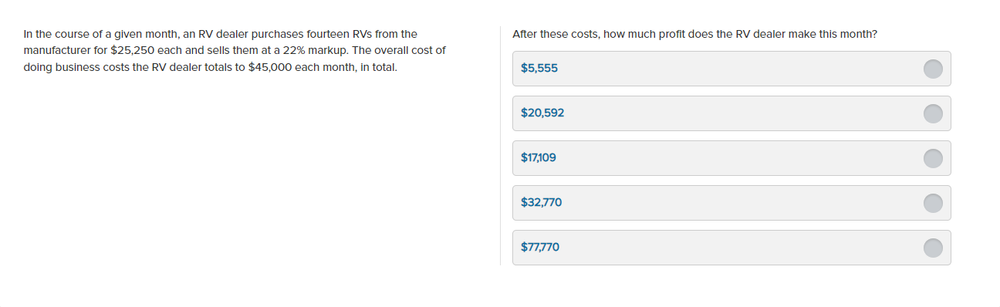Source: SHL

Explanation:
The cost of 14 RVs: 14x \$25,250 = \$353,500
Total cost = \$353,500 + \$45,000 = \$398,500
Mark-up rate = (Revenue – Cost of goods sold) x 100%/ Cost of goods sold = 22%

Therefore, revenue is: \$353,500×0.22 + \$353,500 = \$431,270
The profit RV dealer makes this month: \$431,270 – \$398,500 = \$32,770

So, the correct answer is D.

### Data interpretation

Data interpretation questions ask you to understand, interpret and use graphical and tabular data for calculating a required value. This common question type measures your data analysis and decision-making ability, which is crucial for any business-related role as you regularly face numerical tables and charts. Data interpretation is often found in both versions, especially the interactive test format, where you must drag/drop right on tables and graphs.

Now, let’s look at some examples of this type of question in all versions:

Question 3: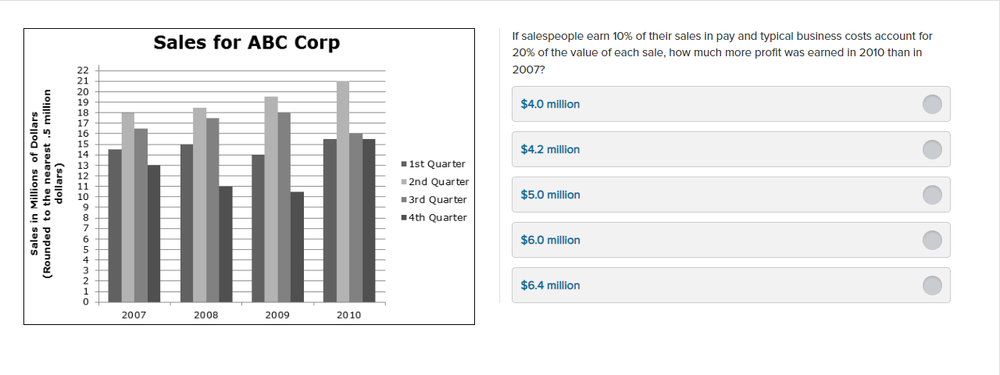Source: SHL

Explanation:
Sales in 2007: 14.5+18+16.5+13 = \$62 million, cost = 20%+10% =30%.
Profit in 2007: 62*(100%-30%) = \$43.4 million
Sales in 2010: 15.5+21+16+15.5 = \$68 million, cost = 20%+10% =30%.
Profit in 2010: 68*(100%-30%) = 47.6 million dollars.
(Profit in 2010 – Profit in 2007) = 47.6-43.4= \$4.2 million.

So, the correct answer is B.

To solve every question in this question type, you can follow 4 simple steps:

Step 1: Determine what type of tables/charts is presented to you. For example, is it a pie chart showing revenue composition, or a graph highlighting the variation in costs?

Step 2: Read the question and determine what type of data you need. Is it an absolute revenue value, or a percentage of sales growth?

Step 3: Locate in the diagram where to provide the information you need. For example, if the question requires you to find the growth rate between 2021 and 2022, you need to look for the indexes for these 2 years on the graph.

Step 4: Apply the information

Knowing these basic steps, let’s practice another data interpretation exercise. This time, we will try out the interactive format.

Question 4:

Explanation:
The average sales profit of each product is presented in the table below: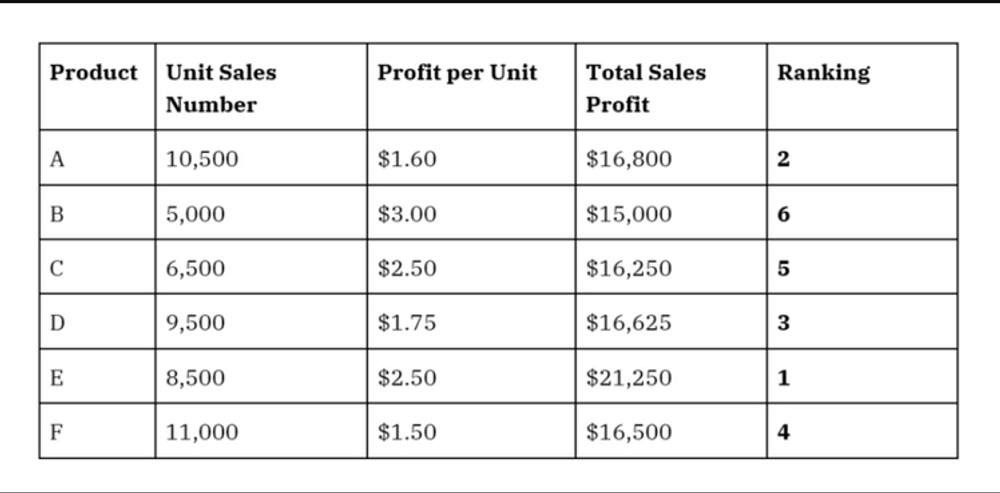Source: SHL

Therefore, products sorted in descending order of sales are E-A-D-F-C-B.